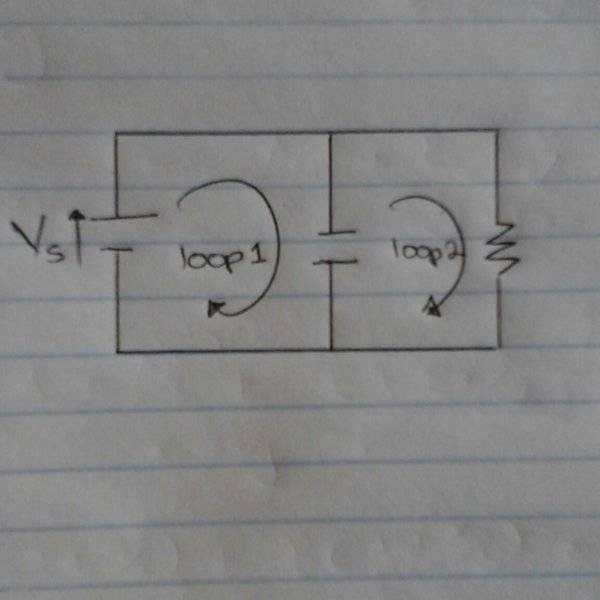# Transient capacitance

When analyzing the transient development of a circuit containing a DC voltage source, capacitor and resistor in parallel, how might one explain the fact that 1. the voltage drop across the resistor changes with that of the capacitor. 2. The first loop containing the capacitor and DC source seem not to obey kirchhoff's voltage law (as the ideal DC source is fixed but the capacitor p.d changes with time)

Last edited:

anorlunda
Staff Emeritus
I can't understand your questions without a picture. Please sketch one and use the UPLOAD button to post it.#### Attachments

jrmichler
Mentor
Your circuit, as drawn, will have infinite current for infinitely short time until the capacitor is charged to the voltage of the voltage source. Then the voltage across the resistor is equal to the voltage across the capacitor.

This will, of course, change if you use a real world voltage source. Real world voltage sources have series resistance, peak current, and transient response characteristics. And real world circuits have wires with finite resistance, capacitance, and inductance. And real world capacitors have internal resistance and inductance.

•Sidney
anorlunda
Staff Emeritus
Here is a good rule you can use. Ohms Law, and Kirckoffs Laes allows us to make models of real world circuits. But whenever they give answers of infinity for voltages or currents, it means that the model is no good and that we must change it in ways such as @jrmichleim said.

CWatters
Homework Helper
Gold Member
When analyzing the transient development of a circuit containing a DC voltage source, capacitor and resistor in parallel, how might one explain the fact that 1. the voltage drop across the resistor changes with that of the capacitor.

The R and C are in parallel. They are connected between the same two nodes therefore they must have the same voltage.

2. The first loop containing the capacitor and DC source seem not to obey kirchhoff's voltage law (as the ideal DC source is fixed but the capacitor p.d changes with time)

What others have said.

You have identified a situation where a circuit model differs from the real world. In the real world batteries, wires and capacitors all have some internal resistance. If you add those resistors to the circuit you will see it can and does meet KVL.

Most components have unwanted parasitic effects. Capacitors also have some inductance. Inductors have some capacitance. Some resistors have both. Batteries aren't ideal voltages sources. Wires have resistance, capacitance and inductance. The list goes on..

In many cases these so-called parasitics are not significant and can be ignored, but not always. In general you have to watch out at high frequencies or transient conditions, or at high or low currents and voltages.

But whenever they give answers of infinity for voltages or currents, it means that the model is no good and that we must change it in ways such as @jrmichleim said.

Does the loop equation give an answer of infinitely in this case? I think what you get is an undefined equation or more specifically a division by zero. Obviously, the conclusion and action one takes to correct the situation is the same either way.

A system that could give infinity as an answer would be something like: find the quiescent point of ideal constant current source charging an ideal capacitor. (At least I think so. I recall the formal definition of quiescent point as voltages, currents at t=infinity for constant inputs but I might be wrong about that.)